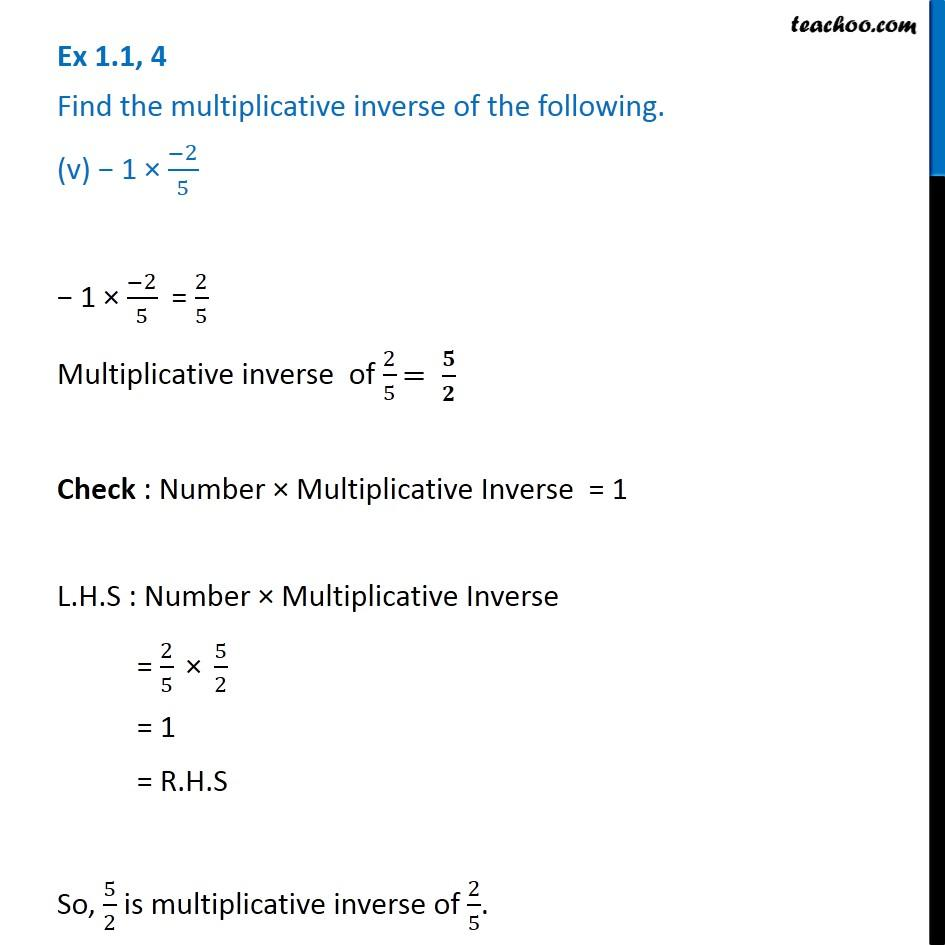Ex 1.1

Chapter 1 Class 8 Rational Numbers
Serial order wiseGet live Maths 1-on-1 Classs - Class 6 to 12

### Transcript

Ex 1.1, 4 Find the multiplicative inverse of the following. (v) 1 ( 2)/5 1 ( 2)/5 = 2/5 Multiplicative inverse of 2/5 Check : Number Multiplicative Inverse = 1 L.H.S : Number Multiplicative Inverse = 2/5 " " 5/2 = 1 = R.H.S So, 5/2 is multiplicative inverse of 2/5.